# GeoGebra excercises

(Export as ggb-files)!!

1. Construct a triangle ∆ABC and its inscribed circle. We can assume that the angles are such that A> B> C. Then construct a second circle that is inscribed in the part of the triangle where C is located. It should touch the first circle and two of the sides of the triangle.

Don't use plagiarized sources. Get Your Custom Essay on
GeoGebra excercises
Get a plagiarism free paper Just from \$13/Page

2. Construct a triangle ∆ABC where all angles are less than 90 degrees. Construct the three heights. The intersections of the heights with the sides form a new triangle, ∆A1B1C1. Send out a beam from A1 towards B1 and reflect it at B1 (angle of incidence is = angle of incidence). Where does the outgoing beam intersect the triangle? Finally, reflect this as well. Conclusion?

3. Five points U1, U2, A, B, C determine a conic section. Choose five points in the plane, no three on a straight line and give them the designations U1, U2, A, B, C. These five points will be on the cone section. Draw the lines q = AB and p = AC and form the point F = U1B U2C. F is thus the intersection of lines U1B and U2C. A sixth point X on the conic section can now be formed as follows: Send out a beam from U1, it intersects the line p at Y1. Then draw the line Y1F and see where it intersects the line q. Call this new point Y2. Finally, pull the beam U2Y2. Our searched point is X = U1Y1 U2Y2. Now change the beam from U1 and see how X changes. Have the trace on function enabled so you see the trace of X. Was it an ellipse, a hyperbola or maybe a parabola? Move your five original points and see if you create other conic sections.

4. Solve problem 8 in the textbook with the help of GG. The figure you are going to draw is given by the following text: You want to measure the distance between two points A and B in the terrain. However, it is impossible to go straight between the two points. Instead, first follow a path from A that forms 50 degrees with AB until you reach a point P. AP = 150 meters. At P, turn 20 degrees towards B along another path until you reach another point Q. P Q = 50. From Q you then turn 40 degrees on a path that leads directly to B. All paths are straight lines. Determine AB.

5. Three circles C1, C2 and C3 all touch each other (all with all) and each touches 2 on each of the following pages in a circumscribed square. Draw such a figure.

6. Place two points on the y-axis. A at (0.1) and B higher up. Now find 2 circles C1 and C2 with midpoints on the x-axis such that C1 should go through A and C2 through B. In addition, the keys in both cases should form 45 degrees with the y-axis. The midpoint of C1 shall have a positive x-coordinate and the midpoint of C2 shall have a negative x-coordinate. C1 and C2 intersect at a point C in the first quadrant. Calculate the angle, γ, between the keys at the point of intersection. You have now constructed a so-called hyperbolic triangle with angles 45 degrees, 45 degrees and γ. Now move point B so that γ comes as close to 45 degrees as possible.

7. Three circles, C1, C2, C3, are disjoint, which means that they are separated (no common points) and they have radii 1,2 and 3. Find the inscribed circle, C, which is tangent to all three circles (all three the circles are thus outside C). Here you need to use the hyperbolic tool. When two circles touch each other, a straight line runs through the midpoints and the tangent point. For a hyperbola, for each point on the hyperbola, the difference in distance to two fixed points is always the same.

8. Two triangles ABC and A1B1C1 that are perspectiveistic from a point O are also perspectiveistic from a line. Illustrate this with GeoGebra. Assume a triangle ABC and a point O outside it. Drag the line OA and select A1 on this line. Then draw the line OB and select B1 on this line and finally the line OC and on the C1. Select all three points on the same page about ∆ABC. Then draw the lines AB and A1B1 they intersect at a point D. The lines AC and A1C1 they intersect at a point E and the lines BC and B1C1 they intersect at a point F. Illustrate with GeoGebra that D, E and F lie on a line.

9. Select a line l and two points F1 and F2 on this and a third point A outside the line. Draw an ellipse with F1 and F2 as focal points passing through point A. Mirror a line passing through one focal point and A and see that it is reflected in the other focal point. For reflection, you need the key to the ellipse at point A. Then illustrate with the help of GG that the sum of the distances from a point on the edge to the two focal points is constant. You must therefore move around the point and see that the sum does not change. Finally, take a point on the ellipse and send out a beam between the focal points F1 and F2. Do three reflections as above. Move your points and see if you can find a periodic course (think of a billiard ball) with three bounces against the ellipse. The track should therefore look like a V.

10. A tool for dividing an angle is usually called a tomahawk. Take a semicircle with radius r and diameter DB and center O. Extend the diameter one distance r to a point A so that | BA | You also need a normal to DA through B. ∠UV W must be divided into three equal parts. Place V on the normal (on the same side as the semicircle). V U should pass through point A and V W should touch the semicircle. Check with GG that the sections V B and V O divide the given angle into three parts. Move around V on the normal and see that it always applies. If you add a similar tomahawk with the center in A that touches the first tomahawk in B, you can divide an angle into five equal parts. Try!

11. A point P is reflected in the three sides of a triangle. Illustrate in GG that the three mirror points lie on the same straight line then and only when P lies on the circle described for the triangle.

12. Construct (almost) a regular hexagon, heptagon (see the leaf on Moodle). You can draw circles with a radius that you specify.

13. Construct the following irregular pentagon: The distance a = 1, b = d = e = 1/2 and c = √ 1 2 (√ 3−1), see figure. It has been found that with the help of such pentagons one can cover the whole planet. If you google Tesselations of the Plane with Pentagons, you will find your figure. Take a few steps in this mosaic. You can mirror points in lines and move points in parallel in a certain direction. You can also determine the color of the pentagons.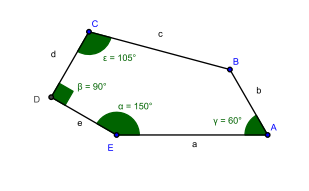14. In a circle with radius r, a circular arc with a center angle of 90 degrees is dashed in the figure below. It is folded over (downwards) and this arc of a circle is solid in the figure. Then a smaller circle is inscribed symmetrically in the figure. Two equal claw-like areas are then formed. Calculate the area of these.

15. Construct an arbitrarily selected triangle ∆ABC where all angles are less than 120 degrees. Then construct an equilateral triangle outward from each side so that you get three new equilateral triangles ∆A1BC, ∆AB1C and ∆ABC1. Show with GG that the lines AA1, BB1 and CC1 are competitive (ie intersect at one point). Call the intersection point P. Illustrate to your students that this point inside the triangle minimizes the sum of the distances to the corners. You can do this by entering another point Q inside the triangle and calculating QA + QB + QC in the input field.

16. Illustrate Ceva’s kit for your students. There is an input field that you must use. You can read about Ceva’s theorem on the internet.

17. Show the nine-point circle to your students. You can read about nine-point circle on the internet.

18. Each triangle ∆ABC has three circumscribed circles. Each of these circles touches one of the sides of the triangle on the outside and the extensions of the other two sides. Construct these three circles. Let A1 be the tangent point on BC, B1 be the tangent point on AC and C1 be the tangent point on AB. Show in GG that lines AA1, BB1 and CC1 are competing (i.e. they intersect at a point).

19. For a given distance AB experiment describe the amount of all possible in-centers for triangles ∆ABC. In-center is the center of the inscribed circle in the triangle. You get to have the trace on function on when you move around point C. Come up with a hypothesis and try to argue for it with the help of GG.

20. In a circle with radius r, a circular arc with a center angle of 90 degrees is dashed in the figure below. It is folded over (downwards) and this arc of a circle is solid in the figure. Then a smaller circle is inscribed symmetrically in the figure. Two equal claw-like areas are then formed. Calculate the area of these.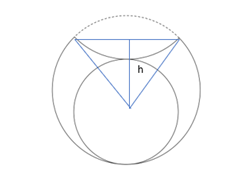21. Start with an ellipse. Select a point A outside the ellipse. Construct the two lines through A that touch the ellipse. Take one of them, it touches the ellipse at a point P. Continue in the direction of the AP for the same distance. Mark this new point with B. So AB = 2AP. Now drag a new key from B and repeat the procedure. This key is tangent to Q and you should therefore continue to C where BC = 2BQ. And so on. Do the iteration 1,2 and 3 more times. Can you find periodic courses of lengths 3,4 and 5?

22. Illustrate Brianchon’s theorem. (Theorem 5.14 in the course book).

23. Construct a figure for the following problem and let GG give an approximate value for the area. Two circles have the same radius and intersect. The angle between the keys of the circles at an intersection is right. The distance between the centers of the circles is 20 (in any unit, eg cm). Determine the area of the area common to the two circles. Here you have to place many points, say 20, on the edge of the area and then use the polygon tool. Click on a point A and then continue to click on point after point along the area. Do not forget to click A at the end. Now you can use the area tool.

24. Let P be a point on the extension of the major axis of an ellipse and denote the midpoint of the key chord to P for Q. The key chord is the chord between the two points on the ellipse obtained when pulling the keys from P to the ellipse. A point P1 lies on a straight line through P parallel to the minor axis of the ellipse. Illustrate with GG that the key to P1 goes through QOrder Now And Get A 20% Discount!
Pages (550 words)
Approximate price: -

Advantages of using our writing services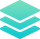Custom Writing From Scratch

All our custom papers are written by qualified writers according to your instructions, thus evading any case of plagiarism. Our team consists of native writers from the USA, Canada, and the Uk, making it convenient for us to find the best to handle your order.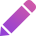Unlimited Free Revisions

If you feel your paper didn't meet all your requirements, we won't stop till it's perfect. You're entitled to request a free revision within 7 days after we submit your paper.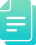Quality Writing In Any Format

If you have issues with citing sources and referencing, you need not worry. Our writers are highly knowledgeable in referencing, including APA/MLA/Havard/Chicago/Turabian and all other formatting styles.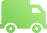All our custom papers are delivered on time, even the most urgent. If we need more time to perfect your paper, we may contact you via email or phone regarding the deadline extension.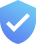Originality & Security

At Master Homework, your security and privacy is our greatest concern. For this reason, we never share your personal information with third parties. We use several writing tools to ensure your paper is original and free from plagiarism.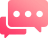Our agents are online 24 hours a day, 7 days a week, and are always ready to serve you. Feel free to contact us through email or talk to our live agents whenever you need assistance with your order.

Try it now!

## Calculate the price of your order

We'll send you the first draft for approval by at
Total price:
\$0.00

How it works?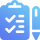Fill in the order form and provide all details of your assignment.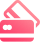Proceed with the payment

Choose the payment system that suits you most.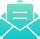Our Services

We work nonstop to see the best client experience.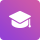## Flexible Pricing

We offer pocket-friendly prices that coincide with the preferred client's deadline.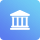Our support team is always ready to ensure vital interaction between you and the writer whenever you need to elaborate on something.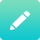Paper Submission

We deliver our papers early within the stipulated deadlines. We are glad to help you if there should be an occurrence of any alterations required.Customer Feedback

Your review, positive or negative, is of great concern to us and we take it very seriously. We are, consequently adjusting our policies to ensure the best customer/writer experience.